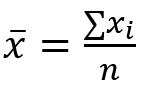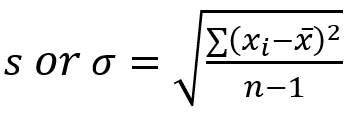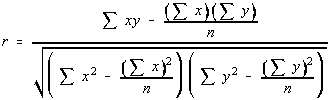# Simple Linear Regression and Multiple Linear Regression explanation and prediction

 Category: Machine Learning Tags:Linear Regression Code Files

## Simple Linear Regression

Simple linear regression is relationship between two variables x, y where a function y = a + b.x can be determined to predict values of y on predictor x.

y = a + b.x

a = intercept, b = slope of line

Suppose we have given data of employee’s experience and their salary:

 Experience (Years) Salary (10000 \$) 2 3 3 3 3.5 3.5 3.5 4 4 4 4.5 4.5 5 6 6 6 7 8 7.5 8

We know here values of x and corresponding y, only we need to find a and b to use the above formula. Below given formula to find a and b:

Where Sy, Sx is Standard deviation of x and y. r is Pearson coefficient. ͞x, ͞y is mean of x and y.

We have already learned about Standard deviation, Mean and Pearson coefficient in previous articles, below are formulas for Mean, Standard deviation and Pearson coefficient:Let’s dump data in a file called Experiencepay.txt

2,3

3,3

3.5,3.5

3.5,4

4,4

4.5,4.5

5,6

6,6

7,8

7.5,8

Create a file called simpleLinearRegression.py and write method to read above file data

```def loadData(filename):
Experience = []
Pay = []
with open(filename) as file:
for row in rows:
exp, pay = row.strip().split(",")
Experience.append(float(exp))
Pay.append(float(pay))
return Experience, Pay```

Above Experience, Pay is X and Y. Pay is proportional to Experience, Experience increases Pay increases. You can plot this data using code:

```here = os.path.dirname(os.path.abspath(__file__))
filename = os.path.join(here, 'Experiencepay.txt')
#experience, pay will be ploted on x, y axis respectively
#plotting scatter plot of actual data
plt.scatter(Experience, Pay, color='red')
plt.xlabel("Experience (Years)")
plt.ylabel("Annual Salary (10000s)")
plt.show()```

Output

Let’s write the method to find a and b using formulas discussed before

```def calculateLinearRegrassionCoffecients(x, y):
a = 0
b = 0
r = 0
n = len(x)
#∑x
sum_x = sum([ele for ele in x])
#∑y
sum_y = sum([ele for ele in y])
avg_x = sum_x/n
avg_y = sum_y/n
#∑x2
sum_x_square = sum([ele**2 for ele in x])
#∑y2
sum_y_square = sum([ele**2 for ele in y])
#∑xy
sum_product_x_y = sum([x[i]*y[i] for i in range(n)])
#pearson coefficient
r = (sum_product_x_y - sum_x*sum_y/n)
r /= math.sqrt((sum_x_square - pow(sum_x, 2)/n)*(sum_y_square - pow(sum_y, 2)/n))
#standard deviation
S_x = math.sqrt(sum([(avg_x - ele)**2 for ele in x])/(n-1))
S_y = math.sqrt(sum([(avg_y - ele)**2 for ele in y])/(n-1))
#slope
b = r*(S_y/S_x)
#intercept
a = avg_y - b*avg_x
return a, b```

Now we have value of a and b, and now  can write a method to predict y:

```def predict(x, a, b):
# y = a + b*x
return (a + b*x)```

Now let’s plot the regression line

```here = os.path.dirname(os.path.abspath(__file__))
filename = os.path.join(here, 'Experiencepay.txt')
#experience, pay will be plotted on x, y axis respectively
#calculating intercept and slope
a, b = calculateLinearRegrassionCoffecients(Experience, Pay)
#prediction line y values for x
y_predict = [predict(x, a, b) for x in Experience]
#plotting scatter plot of actual data
plt.scatter(Experience, Pay, color='red')
#plotting regression line
plt.plot(Experience, y_predict)
plt.xlabel("Experience (Years)")
plt.ylabel("Annual Salary (10000\$)")
plt.show()```

We can predict salary of a new employee

```here = os.path.dirname(os.path.abspath(__file__))
filename = os.path.join(here, 'Experiencepay.txt')
#experience, pay will be plotted on x, y axis respectively
#calculating intercept and slope
a, b = calculateLinearRegrassionCoffecients(Experience, Pay)
x = 12
#prediction line y values for x
y = predict(x, a, b)
print("Salary of {} years experienced person should be {}".format(x, y))```

Output

Salary of 12 years experienced person should be 12.68661971830986

## Multiple Linear Regression

As we seen in simple linear regression there was only one predictor x, in other hand multiple linear regression has more than 1 predictor x1,x2,x3… and we may write formula:

y = a + b1.x1 + b2.x2

Let’s add one more feature called skill level in our data, create file ExpLevelPay.txt

2,2,3

3,3,4.5

3.5,3,4

3.5,5,8

4,4,8

4.5,2,5

5,4,9

6,2,7

7,2,8

7.5,5,9

Create a file called multipleLinearRegression.py and paste below code

```import os
import numpy as np
from sklearn.linear_model import LinearRegression

X = []
Y = []
with open(filename) as file:
for row in rows:
exp,level,pay = row.strip().split(",")
X.append([float(exp),float(level)])
Y.append(float(pay))
return X, Y

here = os.path.dirname(os.path.abspath(__file__))
filename = os.path.join(here, 'ExpLevelPay.txt')
#x is (exp,level) and y is pay
#initializing linear regression
mulReg = LinearRegression()
#training
model = mulReg.fit(X, Y)

#predicting of guy 5 years exp and skill level 5
X1 = [[5,4]]
Y1 = model.predict(X1)
print("Salary of {} years experienced and {} skill level person should be {}".format(X1, X1, Y1))```

Output

Salary of 5 years experienced and 4 skill level person should be [7.64079932]

You can see above code we used sci-kit here to predict salary using multiple linear regression. We can use this LinearRegression module to train and predict.

 Like0 PeopleNikhil Joshi Ceo & Founder at Dotnetlovers Atricles: 146 Questions: 16 Given Best Solutions: 16 *

Login via:x